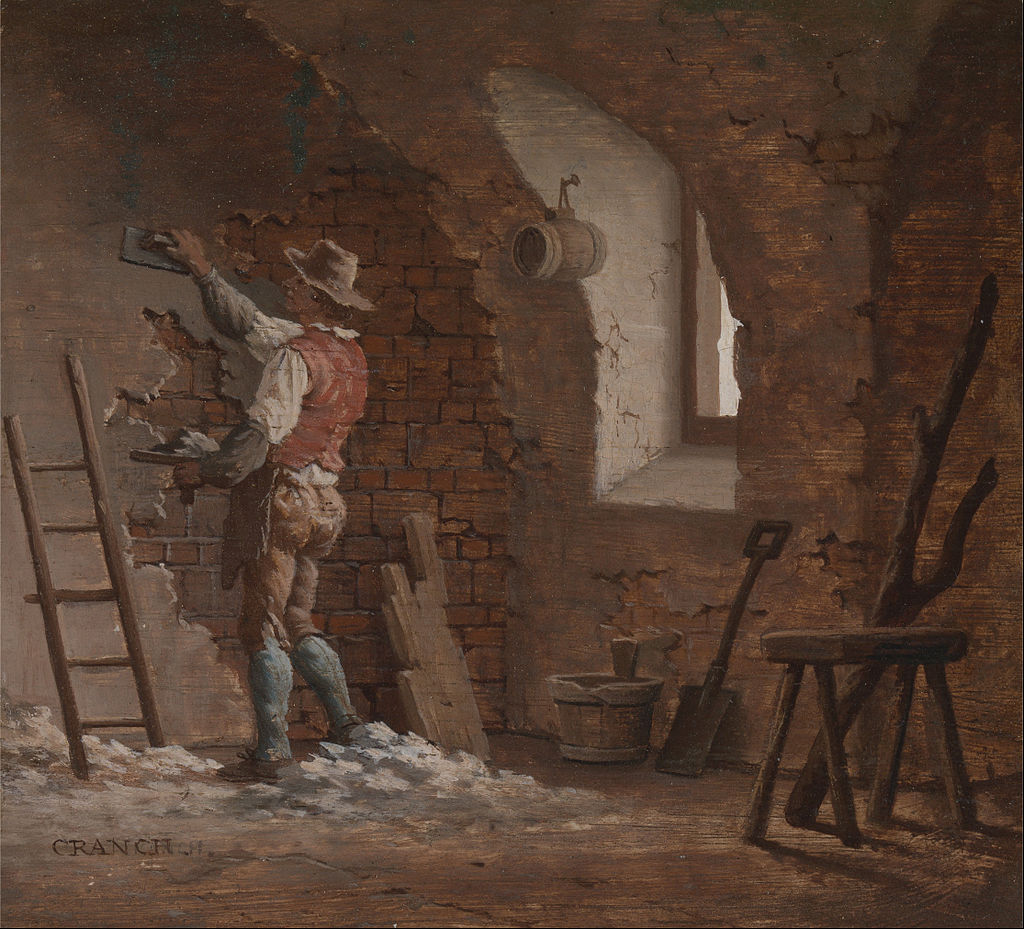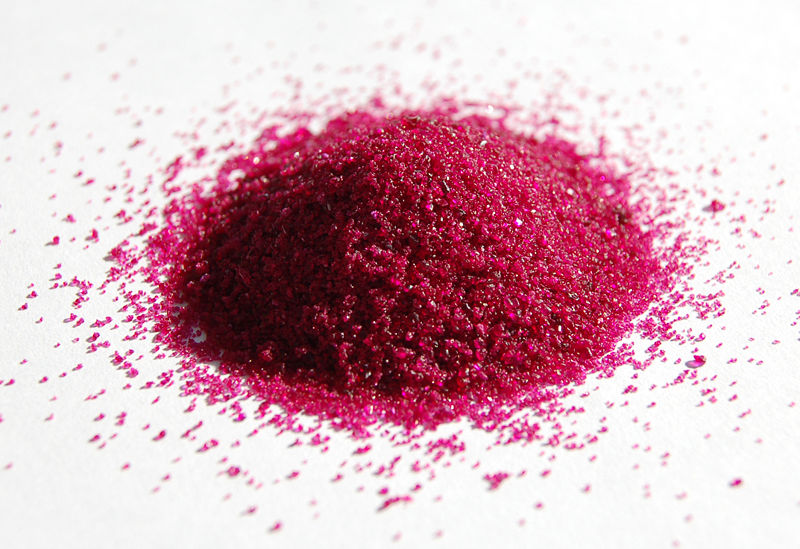Sarah Taylor

HS-PS1-1

# Percent Of Water In A Hydrate Study Guide

A hydrate is a chemical in which each formula unit contains a single or even more water molecules attached to it.

Table of content

# Introduction

We have all seen sculptures like these in our day-to-day lives, either in a museum or a historical place. Did you know that these sculptures are made up of plaster of Paris? If you go to the hardware store and ask for Plaster of Paris, the shopkeeper will give you a bag of white powder. Now you must be wondering how a powder becomes so hard and strong enough to be sculpted into a statue. It is possible through a process called "setting of plaster." Anhydrous POP is in powder form, but the hydrated form is a hard solid that can be shaped into any form we like.Source

That being said, let’s learn in detail about different hydrates and their dependence on water content (percentage water in hydrate).

## PERCENTAGE OF WATER IN A HYDRATE

• Any substance having water in the form of H2O molecules, generally but not necessarily with defined water content in the mixture, is referred to as a hydrate. Hydrates are crystalline materials that shed their essential structures when the bound water is removed.

• The exclusions are zeolites (aluminum silicate minerals or substituted derivatives that contain water indefinitely), comparable clay minerals, specific clays, and metallic oxides, which already have varying percentages of water in their hydrated configurations. Zeolites forfeit and acquire water transiently with no or little structural change.

• Hygroscopic or deliquescent compounds naturally absorb moisture from the atmosphere to create hydrates. Efflorescent materials shed water of hydration or crystallization to become un-hydrated (anhydrous) substances. Water absorption and release (by heating, reducing pressure, or other mechanisms) are often reversible events associated with color changes.

• Transition metal-containing ionic compounds are frequently brightly colored. It's interesting to note that the hydrated phase of a chemical is usually a different hue than that of the amorphous state, which contains no water.Source

## STEPS TO FINDING THE FORMULA OF A HYDRATE

1. Calculate the volume of water that has escaped the compound:

It enables us to calculate the quantity of water in the hydrate and the weight of the anhydrate. We achieve this by deducting the anhydrate's mass from the hydrate's mass. It is the same as the volume of water.

2. Convert the amount of water in liters to moles.

To acquire moles of water, divide the quantity of water by the molecular mass of water.

3. Convert the remaining weight of anhydride to moles.

To determine the moles of anhydrate, divide the weight of anhydrous by the molar mass of anhydrate.

4. Determine the mole ratio of water to anhydrate.

In most cases, you'll have more fluids than anhydrate; therefore, divide total moles of water by the mole of anhydrate. It is how you find out what your mole ratio is.

5. To construct the formula, just use the mole ratio.

Example:

The water was driven off from a 15.67 g sample of a hydrate of magnesium carbonate by heating without decomposing the carbonate. We reduced the mass to 7.58 grams. How do you calculate hydrate's formula?

Solution:

1) Calculate how much water has been driven off:

15.67 − 7.58 = 8.09 grams of water

2) Calculate moles of magnesium carbonate and water:

MgCO3 ---> 7.58 grams / 84.313 grams/mol = 0.0899 mol

H2O ---> 8.09 grams / 18.015 grams/mol = 0.449 mol

3) Calculate the whole number molar ratio:

MgCO3 ---> 0.0899 mol / 0.0899 mol = 1

H2O ---> 0.449 mol / 0.0899 mol = 5

MgCO3 · 5H2O

# Conclusion

• A hydrate is a chemical in which each formula unit contains a single or even more water molecules attached to it.
• Hygroscopic or deliquescent compounds naturally absorb moisture from the atmosphere to create hydrates.
• Transition metal-containing ionic compounds are frequently brightly colored.

## FAQs:

1. How do you find percent H2O?

The proportion of water in the mixture is calculated by dividing the mass of water lost by the initial amount of hydrate utilized. The percent water is calculated by multiplying this percentage by 100.

2. What is the percent by mass of water in the hydrate?

Percent of water in a hydrate is a water molecule that is stoichiometrically bonded into a crystal, expressed as a percentage by mass. Water is often not directly bound to a metal cation, even if contained within a crystal. Hydrates are crystalline salts that include hydration water.

3. What is the mass of water?

18.01528 g/mol is the mass of water according to standard norms.

We hope you enjoyed studying this lesson and learned something cool about Percent of Water in a Hydrate! Join our Discord community to get any questions you may have answered and to engage with other students just like you! Don't forget to download our App to experience our fun VR classrooms - we promise it makes studying much more fun! �*

## SOURCES:

1. Percent of Water in a Hydrate https://www.ck12.org/c/chemistry/percent-of-water-in-a-hydrate/lesson/Percent-of-Water-in-a-Hydrate-CHEM/ Accessed 18 February 2022
2. Hydrates: Determining the Chemical Formula From Empirical Data https://study.com/academy/lesson/hydrates-determining-the-chemical-formula-from-empirical-data.html Accessed 18 February 2022
3. Hydrate. https://www.britannica.com/science/hydrate Accessed 18 February 2022